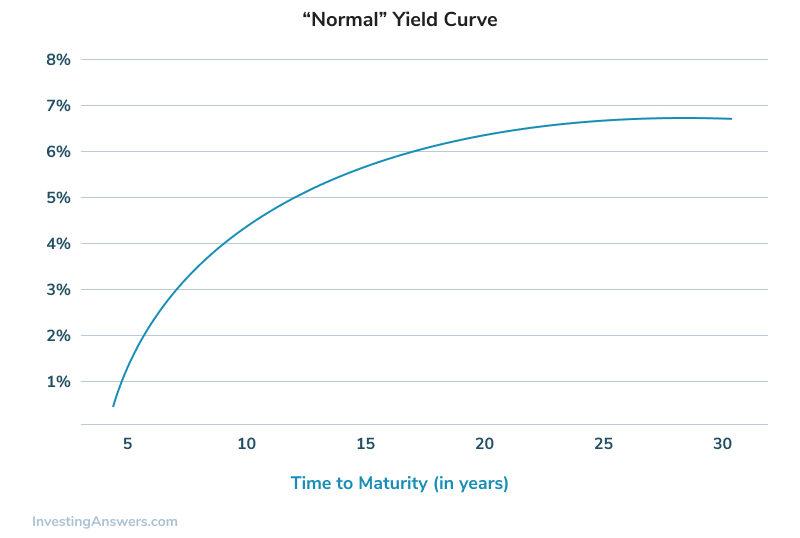## What is a Normal Yield Curve?

Also called a positive yield curve, a normal yield curve is one in which short-term yields are lower than long-term yields.

## How Does a Normal Yield Curve Work?

A yield curve is a graph that plots the yields of similar-quality bonds against their maturities, ranging from shortest to longest. note that the chart does not plot coupon rates against a range of maturities -- that's called a spot curve.

The yield curve shows whether short-term bond yields are higher or lower than long-term bond yields. There are three main types of yield curves. The most common is the positive yield curve. If short-term yields are higher than long-term yields, the curve is called an inverted (or 'negative') yield curve. A flat yield curve exists when there is little or no difference between short- and long-term yields. See the picture below for an example of a normal yield curve.It is important that only bonds of similar risk are plotted on the same yield curve. The most common type of yield curve plots Treasury securities because they are considered risk-free and thus a benchmark for determining the yield on other types of debt.

The shape of the yield curve changes over time, and yield curves are calculated and published by The Wall Street Journal, the Federal Reserve and many financial institutions.

## Why Does a Normal Yield Curve Matter?

Generally, a normal yield curve indicates that investors require a higher rate of return for taking the added risk of lending money for a longer period of time. Many economists also believe that a steep positive curve indicates investors expect higher future inflation (and thus higher interest rates), and that a sharply inverted yield curve means investors expect lower inflation (and interest rates) in the future. A flat curve generally indicates investors are unsure about future economic growth.

Because the yield curve is generally indicative of future interest rates, which are indicative of an economy's expansion or contraction, yield curves and changes in yield curves can convey much information. Changes in the shape of the yield curve also affect a portfolio's return in that they make some bonds more or less valuable relative to other bonds. These concepts are part of what motivates analysts to study yield curves carefully.